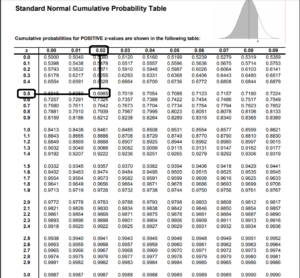# Standard Normal Curve Find Z Score Corresponds 7th Decile

for the standard normal curve, find the z-score that corresponds to the 7th decile.

### Elevate Your Writing with Our Free Writing Tools!

Did you know that we provide a free essay and speech generator, plagiarism checker, summarizer, paraphraser, and other writing tools for free?

Using Z-table:

z-score =  0.52.

Using technology as Excel:

z-score = 0.5244.

## Step by Step Explanation

The z-score for the seventh decile is what we are looking for in this case.

According to math, P(Z z) = 0.70

Z-table use:

Given that the probability closest to 0.7000 is 0.6985

0.52 is the result for 0.6985.